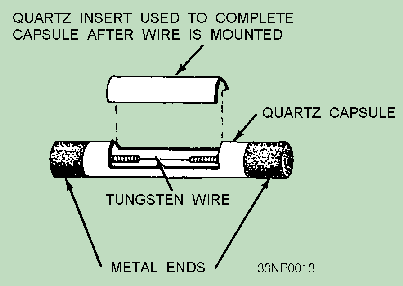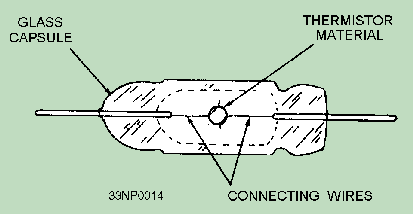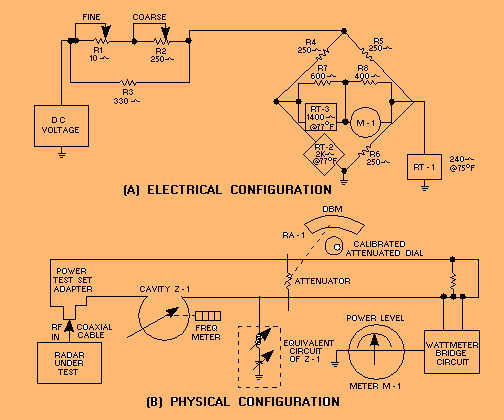resistance as the dissipated power rises. The thermistor decreases in resistance as the power increases. In either case, resistance is measured before and after the application of rf power. If the same change in resistance is then produced by a variable dc source of power, then the rf power is equal to the measured dc power.">Custom SearchIn the UHF and SHF frequency ranges, accurately measuring the voltage, current, and resistance is difficult. These basic measurements can vary greatly, depending on where in the circuit the measurements are made. They are also affected by small changes in parts placement in the vicinity of tuned circuits. To measure the output of microwave radio or radar transmitters, you can use test instruments that convert rf power to another form of energy, such as light or heat. These instruments can be used to indirectly measure the power. A method used to measure the effect of a resistor load on a stream of passing air can also be used to indirectly measure power. Accurate measurement of large-magnitude power also can be achieved by measuring the temperature change of a water load. The most common type of power meter for use in this frequency range employs a BOLOMETER. Bolometer The bolometer is a loading device that undergoes changes of resistance as changes in dissipated power occur. The two types of bolometers are the BARRETTER and the THERMISTOR. The barretter is characterized by an increase in resistance as the dissipated power rises. The thermistor decreases in resistance as the power increases. In either case, resistance is measured before and after the application of rf power. If the same change in resistance is then produced by a variable dc source of power, then the rf power is equal to the measured dc power. This relationship makes possible the direct calibration of a bridge circuit in units of power. In other words, one condition of balance exists when no rf power is applied; but in the presence of power, a second condition of balance exists because of the resistance changes of the bolometer. It is this change of resistance that is calibrated in power. Q.5 What are the two types of bolometers?BARRETTER. - The construction of a typical barretter is shown in figure 2-2. The fine wire (usually tungsten) is extremely small in diameter. This thin diameter allows the rf current to penetrate to the center of the wire. The wire is supported in an insulating capsule between two metallic ends, which act as connectors. Because of these physical characteristics, the barretter resembles a cartridge-type fuse. The enclosure is a quartz capsule made in two parts. One part is an insert cemented in place after the tungsten wire has been mounted. In operation, the barretter is matched to the rf line after power is applied. Figure 2-2. - Typical barretter.THERMISTOR. - A high degree of precision is made possible by the thermistor; therefore, it is widely used. Figure 2-3 shows the typical construction of a bead-type thermistor. The negative-temperature coefficient comes from the use of a semiconductor as the active material. Notice that the active material is shaped in the form of a bead. It is supported between two pigtail leads by connecting wires. The pigtail ends are embedded in the ends of the surrounding glass capsule. Figure 2-3. - Bead-type thermistor.The negative-resistance temperature coefficient of thermistors is desirable. This is because excessive power has the effect of changing the resistance of the thermistor to an extent that causes a pronounced rf mismatch. The resulting decrease in power transfer reduces the likelihood of burnout. Thermistor Bridge Figure 2-4, views A and B, is an example of a THERMISTOR BRIDGE used for rf power measurements. A thermistor bridge circuit includes other thermistor elements, referred to as compensating thermistors. These thermistors respond to fluctuations in ambient temperature so that the bridge balances and calibration are maintained over a wide temperature range. Compensating thermistors are usually in disc form so that they can be mounted on a flat metal surface, such as a chassis or a waveguide. The thermistor bridge in view B is located in the terminating section of a waveguide and contains RT-2, a bead thermistor, and two compensating thermistors, RT-1 and RT-3, on the outside of the waveguide (view A). RA-1 in view B, a calibrated attenuator, controls the amount of rf energy applied to RT-2. Figure 2-4. - Thermistor bridge.Before power is applied, R1 and R2 in view A of figure 2-4 are used to adjust the current through RT-2. When the resistance of RT-2 reaches the equivalent parallel resistance of R6 and RT1 (122.4 ohms), the bridge is balanced. Meter M-1 reads 0 at this time. The rf signal being measured is connected to the test set and applied via the calibrated attenuator to RT-2. This causes the temperature of RT-2 to increase, thus reducing its resistance. The bridge becomes unbalanced, causing meter M-1 to deflect an amount proportional to the decrease in resistance of RT-2. Meter M-1, because of the operation of RT-2, reads average power. Q.6 As the dissipated power increases, what effect does this have on the resistance of a thermistor?Integrated Publishing, Inc. - A (SDVOSB) Service Disabled Veteran Owned Small Business